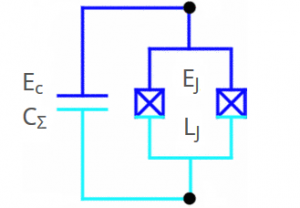# Anton Potočnik

at imec

# Transmon Qubit Calculator

With this calculator you can convert between charging energy (Ec) and total capacitance (CΣ), between Josephson energy (EJ), Josephson inductance (LJ), junction critical current (Ic) or room temperature junction resistance (R), and from charging and Josephson energies calculate relevant qubit transition frequencies.

#### Al Josephson Junction/SQUID

 Ec/2π = GHz CΣ = fF
 EJ/2π = GHz LJ = nH Ic = nA R = Ω

#### Qubit

 ωg-e/2π = GHz ωe-f/2π = GHz ωg-f/2/2π = GHz α/2π = GHzSchematic of a transmon qubit.

#### Parameter Description

 Ec Charging energy CΣ Total capacitance EJ Junction/SQUID Josephson energy LJ Junction/SQUID Josephson inductance Ic Junction/SQUID critical current R Junction/SQUID room temperature resistance for 30/40 nm thin Al films ωg-e Maximal qubit ground (g) to first excited state (e) transition frequency ωe-f Maximal qubit first (e) to second excited state (f) transition frequency ωg-f Maximal qubit ground (g) to second excited state (f) transition frequency α Qubit anharmonicity == Ec

#### Formulas$E_c = \frac{e_0^2}{2C_\Sigma}$$\Phi_0 = 2.067834\cdot10^{-15}\,\mathrm{Wb}$$E_J = \left(\frac{\Phi_0}{2\pi}\right)^2\frac{1}{L_J}$$\Delta = 176\cdot10^{-6}\,\mathrm{V}$$E_J = \frac{\Phi_0}{2\pi}I_c$$e_0 = 1.60218\cdot10^{-19}\,\mathrm{As}$$I_c = \frac{\pi\Delta}{2 R}$$h = 2\pi\hbar = 6.62607 \cdot10^{-34}\,\mathrm{Js}$$L_J = \frac{\Phi_0}{2\pi}\frac{1}{I_c}$$\hbar\omega_{ge} = \sqrt{8 E_c E_J}-E_c$$\hbar\omega_{ef} = \sqrt{8 E_c E_J}-2E_c$$\hbar\omega_{gf}/2 = \sqrt{8 E_c E_J}-1.5E_c$

Tagged under: